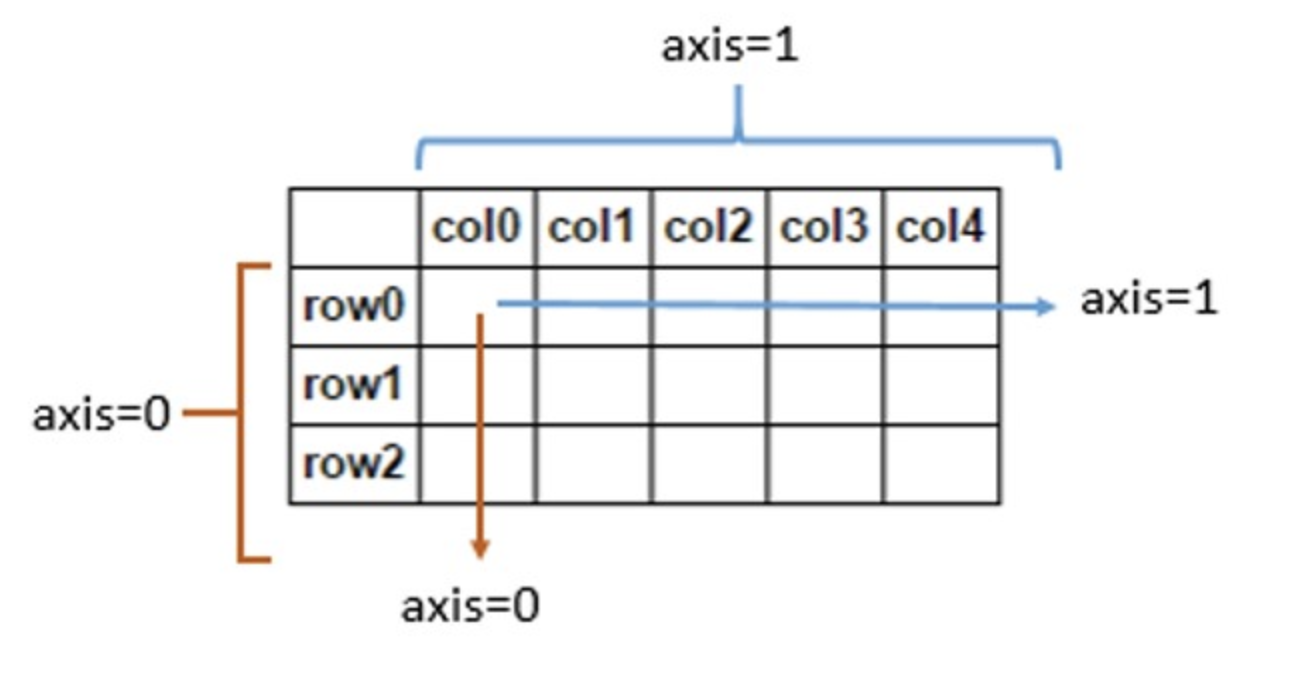# 如何理解pandas中的axis参数

• 为什么
df.drop(index, axis=0)，是去掉某一行数据；
df.drop(col_name, axis=1)，是去掉某一列的数据？
• 为什么
df.sum(axis=0)，是求每的数据之和；
df.sum(axis=1)，是求每的数据之和？

#### 答疑：• axis=0、axis=index，指的是遍历每个index、行号，即在纵向上遍历每列，所以做sum()、mean()等运算时，是对每列数据做操作，而drop(index, axis=0)，传入的参数指定了某一行号，所以会在纵向上遍历每列，去掉行号对应位置的数据
• axis=1、axis=columns，指的是遍历每个columns、列名，即在横向上遍历每行，所以做sum()、mean()等运算时，是对每行数据做操作，而drop(col, axis=1)，传入的参数指定了某一列名，所以会在横向上遍历每行，去掉列名对应位置的数据

#### 补充

pandas和numpy对于axis参数的使用是一致的，从numpy官方术语表对于axis的释义可知一二。
Axes are defined for arrays with more than one dimension. A 2-dimensional array has two corresponding axes: the first running vertically downwards across rows (axis 0), and the second running horizontally across columns (axis 1).

#### 参考

©️2019 CSDN 皮肤主题: 大白 设计师: CSDN官方博客